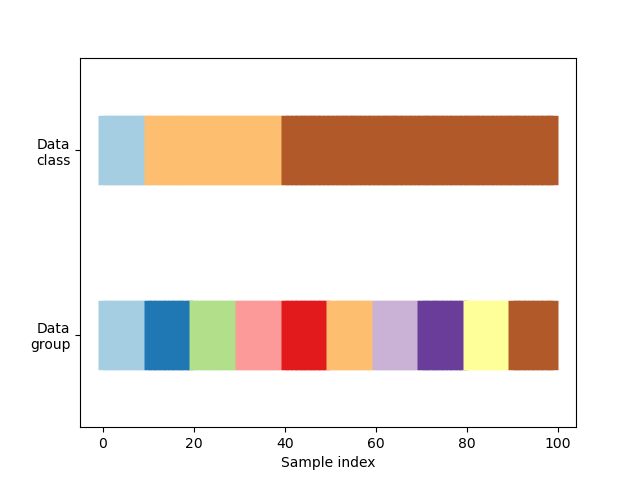# sklearn.model_selection.GroupShuffleSplit¶

class sklearn.model_selection.GroupShuffleSplit(n_splits=5, *, test_size=None, train_size=None, random_state=None)

[源码]

LeavePGroupsOut和GroupShuffleSplit之间的区别在于，前者使用大小为p的唯一组的所有子集进行切分，而GroupShuffleSplit使用由用户确定数量的随机测试集进行切分，每个都有由用户确定的唯一组分数。

n_splits int, default=5

test_size float, int, default=0.2

train_size float or int, default=None

random_state int or RandomState instance, default=None

>>> from sklearn.model_selection import GroupShuffleSplit>>> X = np.ones(shape=(8, 2))>>> y = np.ones(shape=(8, 1))>>> groups = np.array([1, 1, 2, 2, 2, 3, 3, 3])>>> print(groups.shape)(8,)>>> gss = GroupShuffleSplit(n_splits=2, train_size=.7, random_state=42)>>> gss.get_n_splits()2>>> for train_idx, test_idx in gss.split(X, y, groups):...     print("TRAIN:", train_idx, "TEST:", test_idx)TRAIN: [2 3 4 5 6 7] TEST: [0 1]TRAIN: [0 1 5 6 7] TEST: [2 3 4]

get_n_splits(self[, X, y, groups]) 返回交叉验证器中的切分迭代次数
split(self, X[, y, groups]) 生成索引以将数据切分为训练集和测试集。
__init__(self, n_splits=5, *, test_size=None, train_size=None, random_state=None)

[源码]

get_n_splits（self，X = None，y = None，groups = None ）

[源码]

X object

y object

groups object

n_splits int

split（self，X，y = None，groups = None ）

[源码]

X array-like of shape (n_samples, n_features)

y array-like of shape (n_samples,), default=None

groups array-like of shape (n_samples,)

train ndarray

test ndarray

## sklearn.model_selection.GroupShuffleSplit使用示例¶